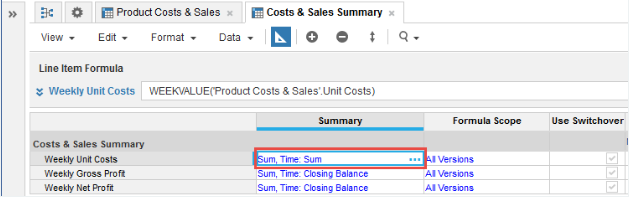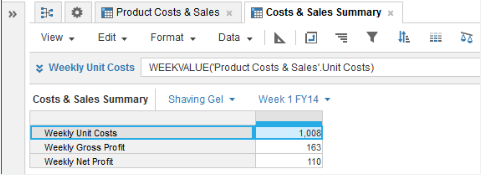1. Calculation functions
2. All Functions
3. Time and Date Functions
4. WEEKVALUEReturns the week value of the source line item or property, according to the Time Summary method set for the source.

## Syntax

`WEEKVALUE(x)`

where:

• x: Line item or property to summarize.

## Format

Input Format Output Format

x Line item - number, Boolean, date, time period, list, text.

Matches the input format.

## Arguments

The function uses the following arguments:

• x: Number, Boolean, date, time period, list item, text.

## Constraints

The function has the following constraints:

• The result formatting must match the source formatting.
• The result must have a timescale applied.
• If the time summary method is none, only numbers get values. Use other time summary methods to get values for Booleans, dates, time periods, list items, and text. See Summary Methods for more information.
• The timescale of the source line item must be Week or Day. If you make the timescale of the source Month, WEEKVALUE returns zero.
• You cannot use WEEKVALUE with Calendar Months/Quarters/Years.

## Excel equivalent

• No Excel equivalent

## Examples

### Sum example

In this example, the time summary method for the Net Profit number-formatted source line item is Sum: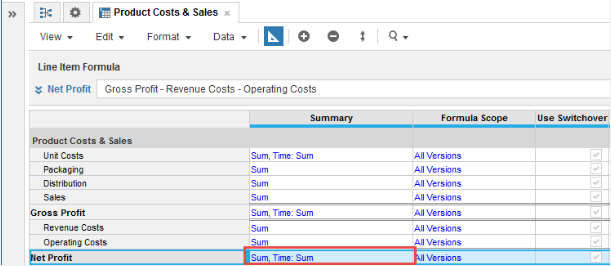You can use WEEKVALUE in a Week Net Profit number-formatted result line item to return the summed values for each week period:

`WEEKVALUE(Net Profit)        `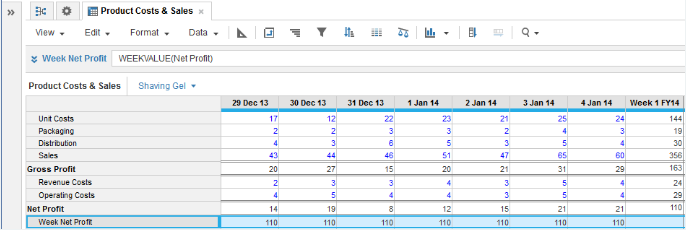### Average example

In this example, the time summary method for the Net Profit source line item is set to Average: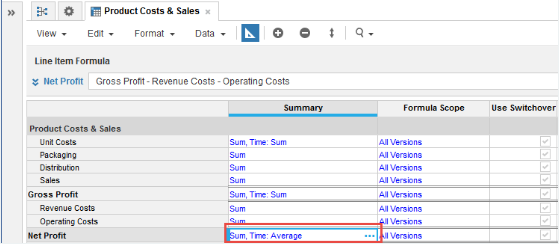You can use WEEKVALUE to return the average daily value for each week period:

`WEEKVALUE(Net Profit)`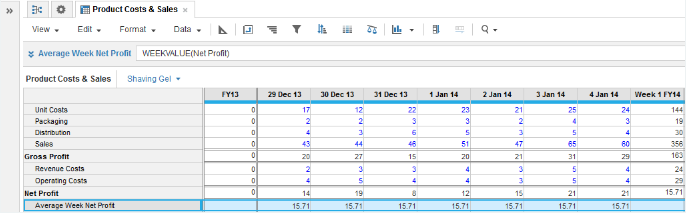### Min example

In this example, the time summary method for the Net Profit source line item is set to Min: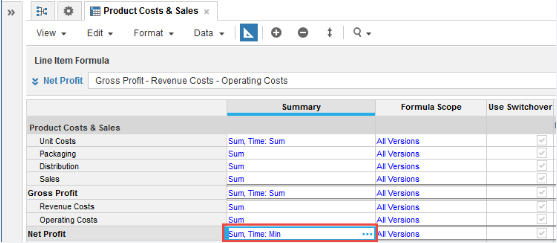You can use WEEKVALUE to return the minimum daily value for each week period:

`WEEKVALUE(Net Profit)        `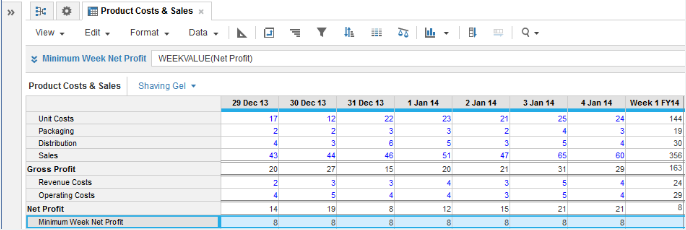### Multiple summary items

In this example, take Product Costs & Sales as a source module for Unit Costs, Gross Profit, and Net Profit figures: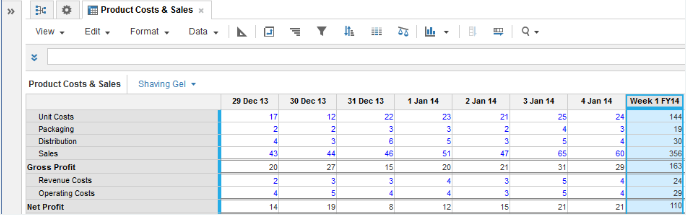The time summary for Unit CostsGross Profit, and Net Profit source line items is set to Sum: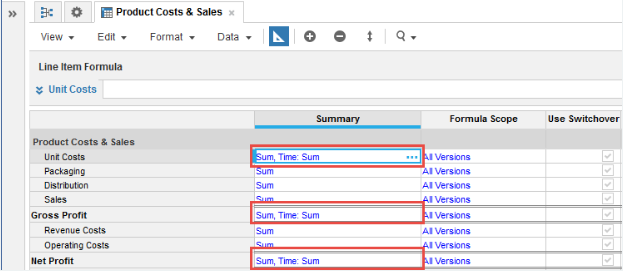In a Costs & Sales Summary results module with a timescale applied, use WEEKVALUE to return Week summary values for Unit CostsGross Profit, and Net Profit:

`WEEKVALUE('Product Costs & Sales'.Unit Costs)        `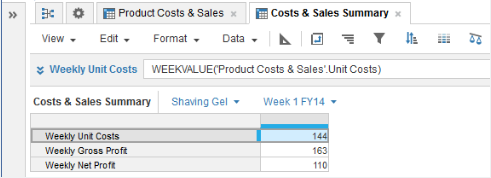Important: Make sure that the time summary method you select for result line items gives you the values that you want. If you change the time summary method for Weekly Unit Costs from Closing Balance to Sum, the value returned for the Week 1 FY14 selection, where the result line item timescale is set to Day, is the multiple of each day's week value: 7 x 144 = 1,008.